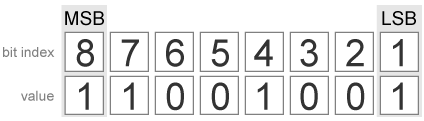# bitget

Get bits at certain positions

## Syntax

``c = bitget(a, bit)``

## Description

example

````c = bitget(a, bit)` returns the values of the bits at the positions specified by `bit` in `a` as unsigned integers of word length 1.```

## Examples

collapse all

Consider the following unsigned fixed-point `fi` number with a value of 85, word length 8, and fraction length 0:

```a = fi(85,0,8,0); disp(bin(a))```
```01010101 ```

Get the binary representation of the bit at position 4:

`c = bitget(a,4);`

`bitget` returns the bit at position 4 in the binary representation of `a`.

Begin with a signed fixed-point 3-by-3 matrix with word length 4 and fraction length 0.

```a = fi([2 3 4;6 8 2;3 5 1],0,4,0); disp(bin(a))```
```0010 0011 0100 0110 1000 0010 0011 0101 0001 ```

Get the binary representation of the bits at a specified position.

`c = bitget(a,fi(2))`
```c=3×3 object 1 1 0 1 0 1 1 0 0 DataTypeMode: Fixed-point: binary point scaling Signedness: Unsigned WordLength: 1 FractionLength: 0 ```

MATLAB® returns a matrix of the bits in position `fi(2)` of `a`. The output matrix has the same dimensions as `a`, and a word length of 1.

Begin with a signed fixed-point vector with word length 16, fraction length 4.

```a = fi([86 6 53 8 1],0,16,4); disp(bin(a))```
```0000010101100000 0000000001100000 0000001101010000 0000000010000000 0000000000010000 ```

Create a vector that specifies the positions of the bits to get.

`bit = [1,2,5,7,4]`
```bit = 1×5 1 2 5 7 4 ```

Get the binary representation of the bits of `a` at the positions specified in `bit`.

`c = bitget(a,bit)`
```c=1×5 object 0 0 1 0 0 DataTypeMode: Fixed-point: binary point scaling Signedness: Unsigned WordLength: 1 FractionLength: 0 ```

`bitget` returns a vector of the bits of `a` at the positions specified in `bit`. The output vector has the same length as inputs, `a` and `bit`, and a word length of 1.

Create a default `fi` object with a value of `pi`.

```a = fi(pi); disp(bin(a))```
```0110010010001000 ```

The default object is signed with a word length of 16.

Create a vector of the positions of the bits you want to get in `a`, and get the binary representation of those bits.

```bit = fi([15,3,8,2]); c = bitget(a,bit)```
```c=1×4 object 1 0 1 0 DataTypeMode: Fixed-point: binary point scaling Signedness: Unsigned WordLength: 1 FractionLength: 0 ```

MATLAB® returns a vector of the bits in `a` at the positions specified by the index vector, `bit`.

## Input Arguments

collapse all

Input array, specified as a scalar, vector, matrix, or multidimensional array of fixed-point `fi` objects. If `a` and `bit` are both nonscalar, they must have the same dimension. If `a` has a signed `numerictype`, the bit representation of the stored integer is in two's complement representation.

Data Types: fixed-point `fi`

Bit index, specified as a scalar, vector, matrix or multidimensional array of `fi` objects or built-in data types. If `a` and `bit` are both nonscalar, they must have the same dimension. `bit` must contain integer values between `1` and the word length of `a`, inclusive. The `LSB` (right-most bit) is specified by bit index `1` and the `MSB` (left-most bit) is specified by the word length of `a`. `bit` does not need to be a vector of sequential bit positions; it can also be a variable index value.

```a = fi(pi,0,8); a.bin```
`11001001`Data Types: `fi`|`single` | `double` | `int8` | `int16` | `int32` | `int64` | `uint8` | `uint16` | `uint32` | `uint64`

## Output Arguments

collapse all

Output array, specified as an unsigned scalar, vector, matrix, or multidimensional array with `WordLength` 1.

If `a` is an array and `bit` is a scalar, `c` is an unsigned array with word length 1. This unsigned array comprises the values of the bits at position `bit` in each fixed-point element in `a`.

If `a` is a scalar and `bit` is an array, `c` is an unsigned array with word length 1. This unsigned array comprises the values of the bits in `a` at the positions specified in `bit`.

## Extended Capabilities

### C/C++ Code GenerationGenerate C and C++ code using MATLAB® Coder™.